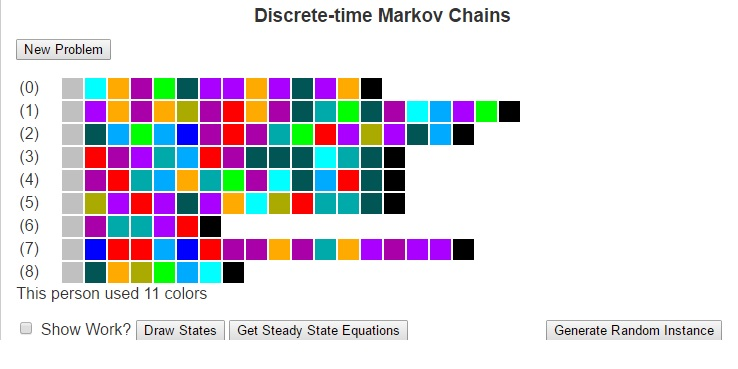Discrete-time Markov ChainsMuch of how we interact with life could be described as transitions between states. These states could be weather conditions (whether we are in a state of “sunny” or “rainy”), the places we may visit (maybe “school”, “the mall”, “the park” and “home”), our moods (“happy”, “angry”, “sad”). There are a number of other ways to model states and even the possibility of infinitely many states.

Markov Chains are based on the principle that the future is only dependent on the immediate past. so for example, if I wished to predict tomorrow’s weather using a Markov Chain, I would need to only look at the weather for today, and can ignore all previous data. I would then compare the state of weather for today with historically how weather has changed in between states to determine the most likely next state (i.e what the weather will be like tomorrow). This greatly simplifies the construction of models.

To use Markov Chains to predict the future, we first need to compute a transition matrix which shows the probability (or frequency) that we will travel from one state to another based on how often we have done so historically. This transition matrix can be calculated by looking at each element of the history as an instance of a discrete state, counting the number of times each transition occurs and dividing each result by the number of times the origin state occurs. I’ll next give an example and then I’ll focus on explaining the Finite Discrete State Markov Chain tool I built using javascript.

Next, I want to consider an example of using Markov Chains to predict the weather for tomorrow. Suppose that we have observed the weather for the last two weeks. We could then use that data to build a model to predict tomorrow’s weather. To do this, lets first consider some states of weather. Suppose that a day can be classified in one of four different ways: {Sunny, Cloudy, Windy, Rainy}. Further, suppose that over the last two weeks we have seen the following pattern.

 Day 1 Sunny Day 2 Sunny Day 3 Cloudy Day 4 Rain Day 5 Sunny Day 6 Windy Day 7 Rain Day 8 Windy Day 9 Rain Day 10 Cloudy Day 11 Windy Day 12 Windy Day 13 Windy Day 14 Cloudy

We can look at this data and calculate the probability that we will transition from each state to each other state, which we see below:

 Rain Cloudy Windy Sunny Rain 0 1/3 1/3 1/3 Cloudy 1/2 0 1/2 0 Windy 2/5 1/5 2/5 0 Sunny 0 1/3 1/3 1/3

Given that the weather for today is cloudy, we can look at the transition matrix and see that historically the days that followed a cloudy day have been Rainy and Windy days each with probability of 1/5. We can see this more mathematically by multiplying the current state vector (cloudy) [0, 1, 0, 0] by the above matrix, where we obtain the result [1/2, 0, 1/2, 0].

In similar fashion, we could use this transition matrix (lets call it T) to predict the weather a number of days in the future by looking at Tn. For example, if we wanted to predict the weather two days in the future, we could begin with the state vector [1/2, 0, 1/2, 0] and multiply it by the matrix T to obtain [1/5, 4/15, 11/30, 1/6].

We can also obtain this by looknig at the original state vector [0, 1, 0, 0] and multiplying it by T2.

T2 =

1

 3/10 8/45 37/90 1/9 1/5 4/15 11/30 1/6 13/50 16/75 59/150 2/15 3/10 8/45 37/90 1/9

When we multiply the original state vector by T2 we arrive at this same answer [1/5, 4/15, 11/30, 1/6]. This matrix T2 has an important property in that every state can reach every other state.

In general, if we have a transition matrix where for every cell in row i and column j, there is some power of the transition matrix such that the cell (i, j) in that matrx is nonzero, then we say that every state is reachable from every other state and we call the Markov Chain regular.

Regular Markov Chains are important because they converge to what’s called a steady state. These are state vectrs x = [x0, …, xn] such that xTn = x for very large values of n. The steady state tells us how the Markov Chain will perform over long periods of time. We can use algebra and systems of linear equations to solve for this steady state vector.

For the Javascript program I’ve written, I have generated a set of painting samples for a fictional artist. The states are the different colors and the transitions are the colors that the artist will use after other colors. as well as the starting and ending colors. Given this input, we can form a Markov Chain to understand the artist’s behavior. This Markov Chain can then be used to solve for the steady state vector or to generate random paintings according to the artist’s profile. Be sure to check it out and let me know what you think.